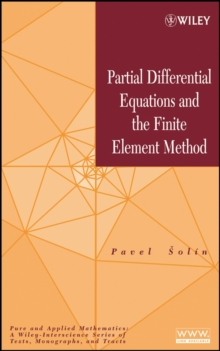Supporting your high street Find out how »
• My AccountPartial Differential Equations and the Finite Element Method Hardback

Part of the Pure and Applied Mathematics: A Wiley Series of Texts, Monographs and Tracts series

Description

A systematic introduction to partial differential equations and modern finite element methods for their efficient numerical solution Partial Differential Equations and the Finite Element Method provides a much-needed, clear, and systematic introduction to modern theory of partial differential equations (PDEs) and finite element methods (FEM).

Both nodal and hierachic concepts of the FEM are examined.

Reflecting the growing complexity and multiscale nature of current engineering and scientific problems, the author emphasizes higher-order finite element methods such as the spectral or hp-FEM.

A solid introduction to the theory of PDEs and FEM contained in Chapters 1-4 serves as the core and foundation of the publication.

Chapter 5 is devoted to modern higher-order methods for the numerical solution of ordinary differential equations (ODEs) that arise in the semidiscretization of time-dependent PDEs by the Method of Lines (MOL).

Chapter 6 discusses fourth-order PDEs rooted in the bending of elastic beams and plates and approximates their solution by means of higher-order Hermite and Argyris elements. Finally, Chapter 7 introduces the reader to various PDEs governing computational electromagnetics and describes their finite element approximation, including modern higher-order edge elements for Maxwell's equations.

The understanding of many theoretical and practical aspects of both PDEs and FEM requires a solid knowledge of linear algebra and elementary functional analysis, such as functions and linear operators in the Lebesgue, Hilbert, and Sobolev spaces.

These topics are discussed with the help of many illustrative examples in Appendix A, which is provided as a service for those readers who need to gain the necessary background or require a refresher tutorial.

Appendix B presents several finite element computations rooted in practical engineering problems and demonstrates the benefits of using higher-order FEM.

Numerous finite element algorithms are written out in detail alongside implementation discussions.

Exercises, including many that involve programming the FEM, are designed to assist the reader in solving typical problems in engineering and science. Specifically designed as a coursebook, this student-tested publication is geared to upper-level undergraduates and graduate students in all disciplines of computational engineeringand science.

It is also a practical problem-solving reference for researchers, engineers, and physicists.

Information

• Format: Hardback
• Pages: 504 pages
• Publisher: John Wiley & Sons Inc
• Publication Date:
• Category: Numerical analysis
• ISBN: 9780471720706

£134.00

£118.75

on all orders

Pick up orders

from local bookshops

£41.99

£37.79

£106.00

£94.45